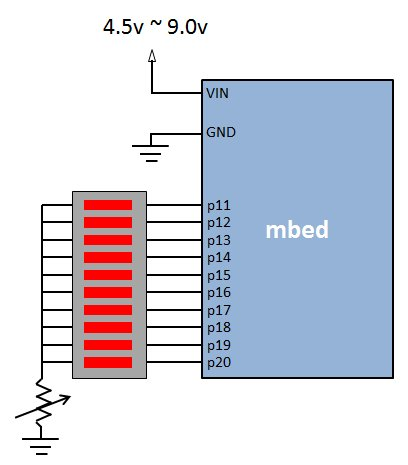## BusOut

### Example 1

A LED light bar is connected from `p11` to `p20` of a mbed, The program moves a light bar from the 1st position to the 10th position.```#include "mbed.h"

#define WAIT_SECOND 0.1

//  LSB                                          MSB
BusOut      Bar(p20, p19, p18, p17, p16, p15, p14, p13, p12, p11);
DigitalOut  Led(LED1);

int main() {
Led = 0;
while(1) {
// Shift from LSB to MSB
for (int i = 0; i < 10; i++) {
Led = !Led;
Bar = 1 << i;
wait(WAIT_SECOND);
}
}
}
```

### Example 2

Let's try to make the light bar bound back from the edges.

```#include "mbed.h"

#define WAIT_SECOND 0.1

//  LSB                                          MSB
BusOut      Bar(p20, p19, p18, p17, p16, p15, p14, p13, p12, p11);
DigitalOut  Led(LED1);

int main() {
Led = 0;
while(1) {
// Shift from LSB to MSB
for (int i = 0; i < 10; i++) {
Led = !Led;
Bar = 1 << i;
wait(WAIT_SECOND);
}

// Shift from MSB to LSB
for (int i = 0; i < 10; i++) {
Led = !Led;
Bar = 0x0200 >> i;
wait(WAIT_SECOND);
}
}
}
```

However, the light bar stops at the both edges for an extra time each. Let change the index in the `for` loop to start from 1 instead of 0.

```#include "mbed.h"

#define WAIT_SECOND 0.1

//  LSB                                          MSB
BusOut      Bar(p20, p19, p18, p17, p16, p15, p14, p13, p12, p11);
DigitalOut  Led(LED1);

int main() {
Led = 0;
while(1) {
// Shift from LSB to MSB
for (int i = 1; i < 10; i++) {
Led = !Led;
Bar = 1 << i;
wait(WAIT_SECOND);
}

// Shift from MSB to LSB
for (int i = 1; i < 10; i++) {
Led = !Led;
Bar = 0x0200 >> i;
wait(WAIT_SECOND);
}
}
}
```

However, during the first start, the LSB pin (p20) is not switched on. Let's fix this:

```#include "mbed.h"

#define WAIT_SECOND 0.1

//  LSB                                          MSB
BusOut      Bar(p20, p19, p18, p17, p16, p15, p14, p13, p12, p11);
DigitalOut  Led(LED1);

int main() {
Led = 0;
Bar = 1; wait(WAIT_SECOND); // Start from LSB first
while(1) {
// Shift from LSB to MSB
for (int i = 1; i < 10; i++) {
Led = !Led;
Bar = 1 << i;
wait(WAIT_SECOND);
}

// Shift from MSB to LSB
for (int i = 1; i < 10; i++) {
Led = !Led;
Bar = 0x0200 >> i;
wait(WAIT_SECOND);
}
}
}
```

### Example 3

Let's modify the program in Example 3 to have the so-called Cyclon or Knight Rider effect

```#include "mbed.h"

#define WAIT_SECOND 0.1

//  LSB                                          MSB
BusOut      Bar(p20, p19, p18, p17, p16, p15, p14, p13, p12, p11);
DigitalOut  Led(LED1);

int main() {
Led = 0;
Bar = 3; wait(WAIT_SECOND); // Start from LSB first
while(1) {
// Shift from LSB to MSB
for (int i = 1; i < 10; i++) {
Led = !Led;
Bar = 3 << i;
wait(WAIT_SECOND);
}

// Shift from MSB to LSB
for (int i = 1; i < 10; i++) {
Led = !Led;
Bar = 0x0300 >> i;
wait(WAIT_SECOND);
}
}
}
```